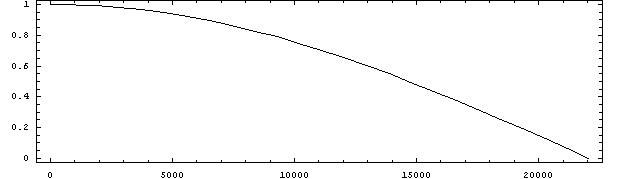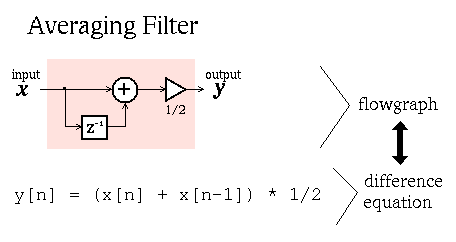# Averaging Filter

Here is the frequency response of the Averaging filter when the sampling rate it 44100 Hz:Here is the definition of the averaging filter both as a flowgraph and as a difference equation. Note that the two description of the filter are equivalent. If you know one form, then you can create the other form.# Exercises

1. Use the osc program to generate a chirp which is a continuously rising (or falling) sinewave pitch.
```   osc -d 5.0 -f "0 0 1 22050" chirp.wav
```

2. Apply the average filter to the chirp and then apply the difference filter to the chirp and listen to the output.
```   average chirp.wav chirpA.wav
```
Listen to the output.

3. Use the subtract program to listen to what the filter removed from the input sound:
```   subtract chirp.wav chirpA.wav chirpAdiff.wav
```

4. Use the whitenoise program to generate some noise:
```   whitenoise -d 3 whitenoise.wav
```
Do similar experiments as with the chirp sound with the whitenoise input to the filter.
5. What is the relationship between the whitenoise input and the chirp input?

6. What is a linear filter?

7. Choose a frequency on the frequency response plot for the filter above. Generate a sinewave at that frequency with the osc program with an amplitude of 1.0. Filter the sinewave. Use the sndpower program to measure the amplitude of the output sinewave.

8. Listen to several sinewave inputs and outputs at different frequencies. Notice the pitch of the sinewaves. How do the pitches of the sinewave change as they go through the filter? How do the amplitudes of the sinewave change as they go through the filter?

9. Listen to the same sound inputs and outputs going through the difference filter.

10. Take the output from the averaging filter and put it into the difference filter. How does the output sound. How would you describe the frequency response. It is probably too hard to describe completely because you cannot hear the very highest part of the range.

11. Listen to the effect of switching the difference and averaging filter order, so that the difference part is done first. How does this change the final output sound?

12. Extra Credit: Use the sndpower program and the chirp sound to generate a frequency response graph similar to the one at the top of the page.
```

```